# Rigid Transformation – Definition, Types, and Examples

The rigid transformation is a classification of transformations. From its name, rigid transformation retains the physical characteristics of the pre-image. However, the direction and position of the image may differ.

The three most common basic rigid transformations are reflection, rotation, and translation. These three transformations all preserve the same properties: size and shape. This is also why dilation does not exhibit rigid transformation.

This article breaks down the conditions for rigid transformations. We’ll also show why the three mentioned transformations are examples of rigid transformations. By the end of this discussion, readers will feel confident when working with this concept.

## What Is a Rigid Transformation?

Rigid transformation (also known as isometry) is a transformation that does not affect the size and shape of the object or pre-image when returning the final image. There are three known transformations that are classified as rigid transformations:  reflection, rotation and translation.

Rigid transformations can also be a combination of these three basic transformations.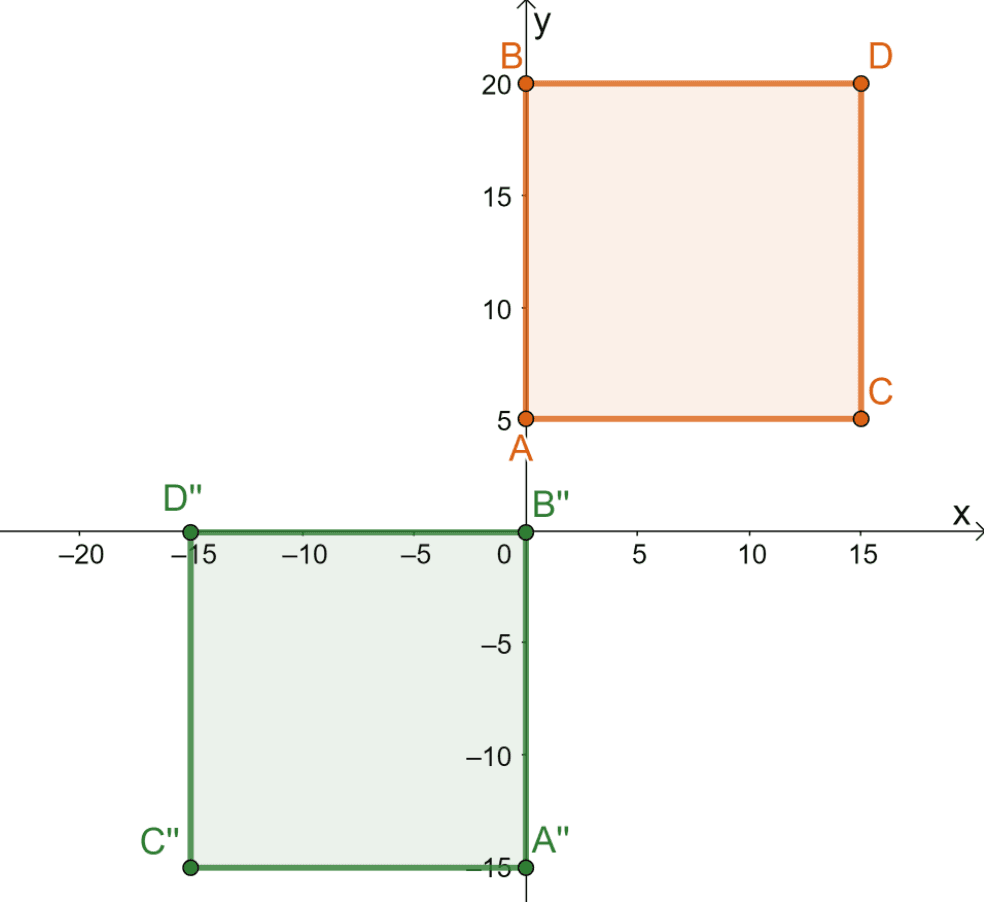Take a look at the pre-image of the square, $ABCD$, and the resulting image $A^{\prime\prime} B^{\prime\prime} C^{\prime\prime}$. Recall that we label the object to be transformed as a pre-image and the resulting object is called the image. As can be seen from transformation, the image retains its pre-image’s shape and size.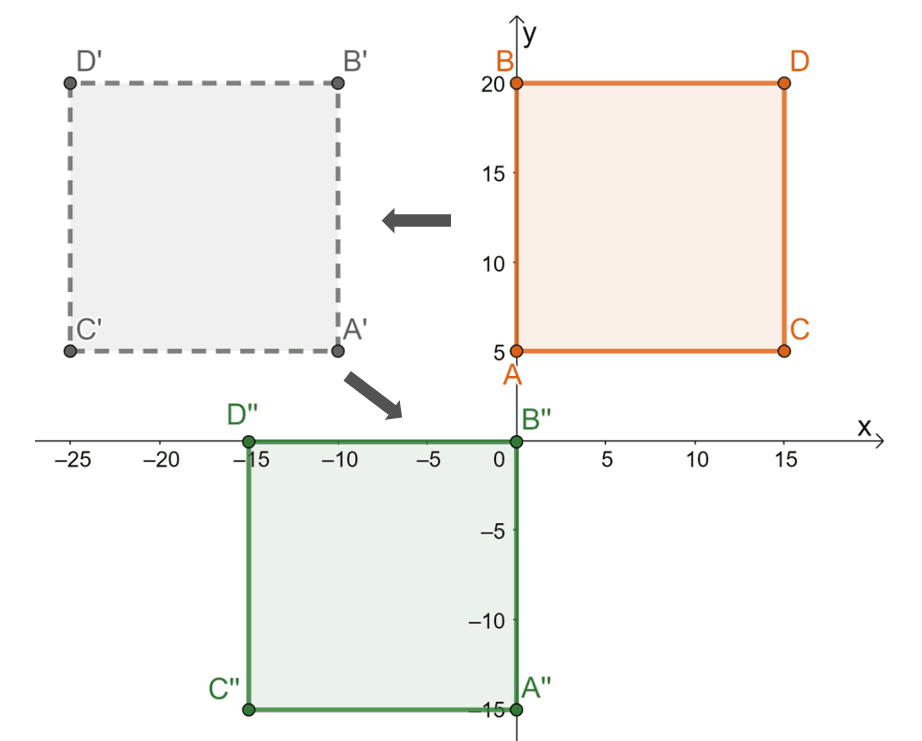This shows that the transformation performed on the square is a rigid transformation. Breaking down the series of transformations performed on the pre-image highlights the story behind the rigid transformation:

• The square $ABCD$ is reflected over the line $x = -5$. The reflected points are $5$ units from the left of the vertical line $x = -5$.
• The reflected square is then translated $10$ units to the right and $20$ units downward.

The series of basic rigid transformations still result in a more complex rigid transformation. This shows that when dealing with rigid transformations, it is important to be familiar with the three basic rigid transformations. This is why it’s essential to have a refresher and understand why they’re each classified as a rigid transformation.

## Rigid Transformation Examples

Some examples of rigid transformations occur when a pre-image is translated, reflected, rotated or a combination of these three.

These three transformations are the most basic rigid transformations there are:

1. Reflection: This transformation highlights the changes in the object’s position but its shape and size remain intact.
2. Translation: This transformation is a good example of a rigid transformation. The image is the result of “sliding” the pre-image but its size and shape remain the same.
3. Rotation: In rotation, the pre-image is “turned” about a given angle and with respect to a reference point, retaining its original shape and size. This makes this transformation a rigid transformation.

It’s time to explore these three examples of basic rigid transformations first. We’ll explore different examples of reflection, translation and rotation as rigid transformations. Once we’ve established their foundations, it will be easier to work on more complex examples of rigid transformations.

### Reflection as Rigid Transformation

In reflection, the position of the points or object changes with reference to the line of reflection. When learning about point and triangle reflection, it has been established that when reflecting a pre-image, the resulting image changes position but retains its shape and size. This makes reflection a rigid transformation.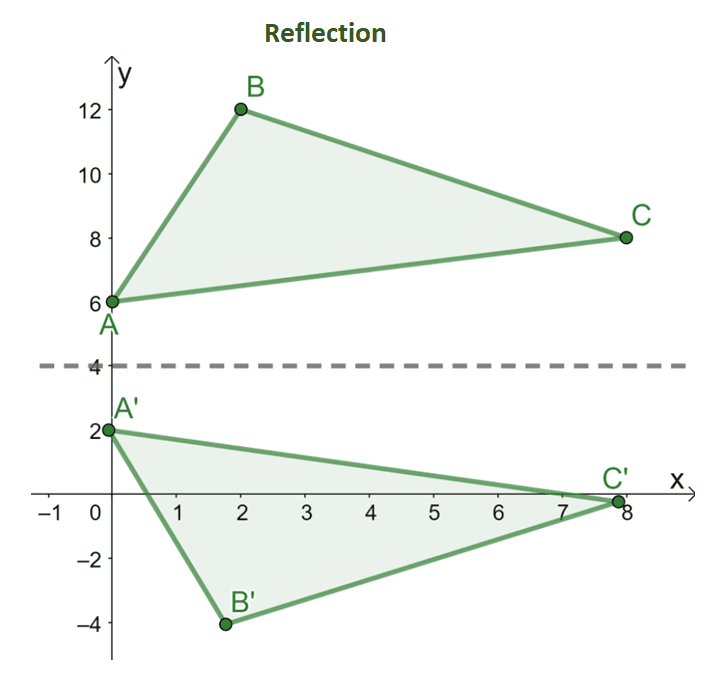The graph above showcases how a pre-image, $\Delta ABC$, is reflected over the horizontal line of reflection $y = 4$. The distances between the vertices of the triangles from the line of reflection will always be the same. In fact, in reflection, the angle measures of the objects, parallelism, and side lengths will remain intact.

However, the orientation of the points or vertices changes when reflecting an object over a line of reflection. The four most common reflections are performed over the following lines of reflection: the $x$-axis, the $y$-axis, $y =x$, and $y =-x$.

This why rules have been established for these types of reflections:

 Reflection Type Coordinates $x$-axis \begin{aligned}(x,y) \rightarrow (x, -y)\end{aligned} $y$-axis \begin{aligned}(x,y) \rightarrow (-x, y)\end{aligned} $y = x$ \begin{aligned}(x,y) \rightarrow (y, x)\end{aligned} $y = -x$ \begin{aligned}(x,y) \rightarrow (-y, -x)\end{aligned}

### Translation as Rigid Transformation

Translation is also a rigid transformation because it simply “moves” the pre-image on a position to construct the final image of the transformation. When translating an object, it is possible to move along the horizontal direction, vertical direction, or even both. Take a look at the translation performed on the triangle $\Delta ABC$.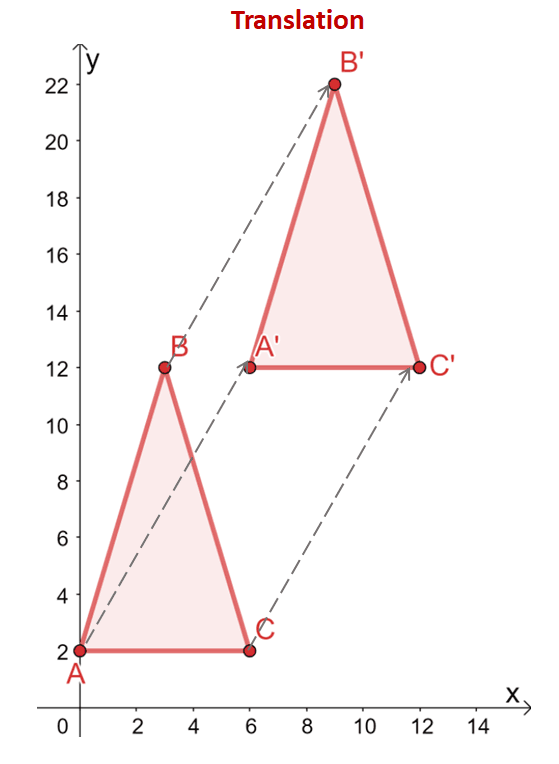The triangle $\Delta ABC$ is translated $6$ units to the right and $10$ units upward. The vertices of the triangle reflect this translation as well: from $(x, y)$, the vertices are translated along with the same horizontal and vertical directions: $(x, y) \rightarrow (x + 6, y + 10)$.

\begin{aligned}A = (0,2) &\rightarrow A^{\prime} = (6,12)\\B = (2,12) &\rightarrow B^{\prime} = (8, 22)\\C = (6 2) &\rightarrow C^{\prime} = (12,12)\end{aligned}

Comparing the two triangles, the shapes and sizes of the two triangles remain intact. The only difference between the pre-image ($\Delta ABC$) and the image ($\Delta A^{\prime}B^{\prime}C^{\prime}$) are their positions. This highlights why translations are classified as rigid transformations.

Use the guide below when working with translations:

 Translation Guide $h$ units to the right$h$ units to the left \begin{aligned}(x,y) &\rightarrow (x+h, y)\\(x, y) &\rightarrow (x-h, y) \end{aligned} $k$ units upward$k$ units downward \begin{aligned}(x, y) &\rightarrow (x, y + k)\\ (x,y) &\rightarrow (x, y – k)\end{aligned} $h$ units to the right, $k$ units upward$h$ units to the left, $k$ units upward \begin{aligned}(x, y) &\rightarrow (x + h, y + k)\\ (x,y) &\rightarrow (x -h, y + k)\end{aligned} $h$ units to the right, $k$ units downward$h$ units to the left, $k$ units downward \begin{aligned}(x, y) &\rightarrow (x + h, y – k)\\ (x,y) &\rightarrow (x -h, y – k)\end{aligned}

### Rotation as Rigid Transformation

In rotation, the pre-image is “turned” for a given angle in either a clockwise or counter-clockwise direction and with respect to a given point. This makes it a rigid transformation because the resulting image retains the size and shape of the pre-images.

Here’s an example of a rotation involving $\Delta ABC$, where it is turned at an angle of $90^{\circ}$ in a counter-clockwise direction and with respect to the origin.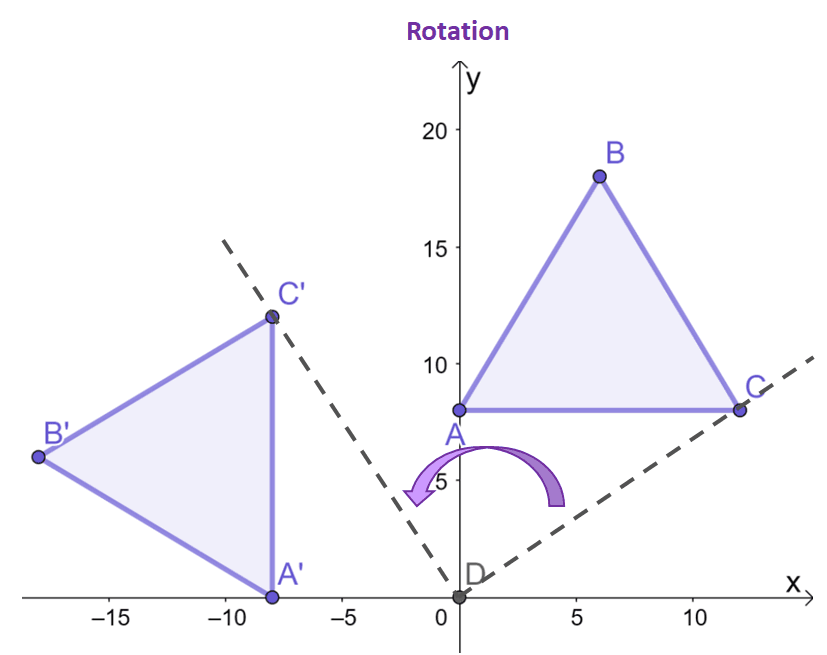Focus on the points, $C$ and $C^{\prime}$, see how with respect to the origin, the resulting point of the image is turned $90^{\circ}$ counter-clockwise?

The two remaining vertices for the image and pre-image will exhibit the same behavior.  As can be observed between the two triangles, $\Delta ABC$ and $\Delta A^{\prime}B^{\prime}C^{\prime}$, have the same size and shape, highlighting its nature as a rigid transformation.

The rules for transformation have been established in the past, so here’s a quick guide when rotating the objects in a counter-clockwise direction and about the origin.

 Rotation Guide (Counter-clockwise Direction) \begin{aligned}90^{\circ}\end{aligned} \begin{aligned}(x, y) \rightarrow (-y, x)\end{aligned} \begin{aligned}180^{\circ}\end{aligned} \begin{aligned}(x, y) \rightarrow (-x, -y)\end{aligned} \begin{aligned}270^{\circ}\end{aligned} \begin{aligned}(x, y) \rightarrow (y, -x)\end{aligned}

Now that we’ve covered all three main examples of rigid transformations, it’s time to use our knowledge to work on more advanced problems involving rigid transformations. When you’re ready, head over to the section below!

### Example 1

Which of the following transformations do not exhibit rigid transformation?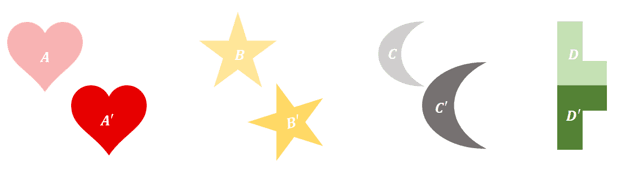### Solution

Observe each pair of pre-image and images then try to describe the transformations applied on each of the objects.

• The size and shape of both $A$ and $A^{\prime}$ are identical. The only difference is that $A^{\prime}$ is the result of translating $A$ to the right and downward.
• Now, focus on $B$ and $B^{\prime}$. The image of $B$ is the result of rotating it $90{\circ}$ to the counter-clockwise direction. In rotation, shape and size are also retained.
• For $C$ and $C^{\circ}$, $C^{\prime}$ clearly is a scaled version of $C$. In fact, $C$ is stretched and translated to find the image $C^{\prime}$.
• $D$ and $D^{\circ}$ are facing opposite each but they both have the same size and shape.

From these observations, it is clear that $A$, $B$, and $D$ exhibit rigid transformations only. However, for $C$ and $C^{\prime}$, since size has changed, they do not exhibit rigid transformations.

### Example 2

The triangle $\Delta ABC$ is graphed on the rectangular coordinate system. The vertices of the triangle have the following coordinates:

\begin{aligned}A &= (2, 2)\\ B&= (8, 4)\\C &= (4, 10)\end{aligned}

If $\Delta ABC$ is translated $10$ units to the left and $2$ units upward, what are the coordinates of $\Delta A^{\prime}B^{\prime}C^{\prime}$? Use the resulting image to confirm that the transformations applied were all rigid.

### Solution

Use the coordinates of $A$, $B$, and $C$ to plot the vertices of $\Delta ABC$ and sketch its figure. To translate $\Delta ABC$ $10$ units to the left and $2$ units upward, subtract $10$ from the $x$-coordinate and add $2$ to each $y$-coordinate.

\begin{aligned}A^{\prime} &= (2 -10, 2 2)\\&= (-8, 4)\\ B^{\prime}&= (8- 10, 4 + 2)\\&= (-2, 6)\\C^{\prime} &= (4 -10, 10+2)\\&= (-6, 12)\end{aligned}

Another way of translating the vertices of $\Delta ABC$ is by manually moving each vertex’s coordinates $10$ units to the left and $2$ units upward as shown below.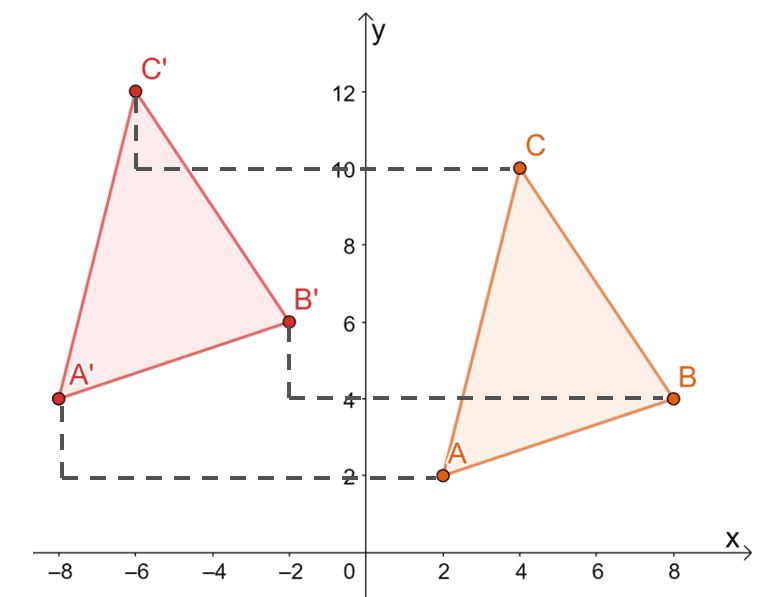Hence, we have the image of $\Delta A^{\prime}B^{\prime}C^{\prime}$ as shown by the graph below. Both methods result in the same image, confirming that we can use both methods.

This means that the vertices of $\Delta A^{\prime}B^{\prime}C^{\prime}$  are $A^{\prime}=(-8, 4)$, $B^{\prime}=(-2, 6)$, and $C^{\prime}=(-6, 12)$.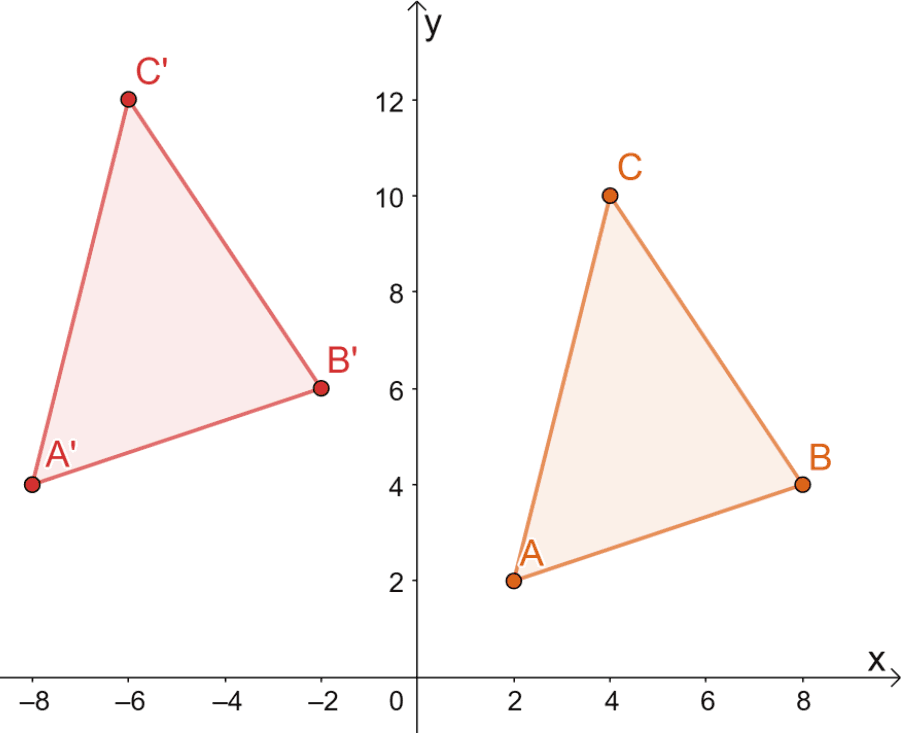From the resulting image, the two triangles share the same size and shape. They only differ by their position, so the only transformations that can be observed are all rigid.

### Practice Question

1. Which of the following transformations do not exhibit rigid transformation?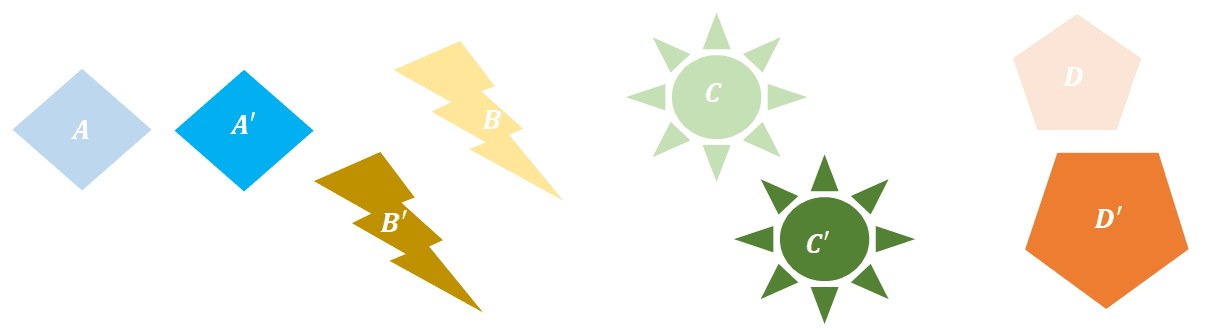A. $B \rightarrow B^{\prime}$
B. $B\rightarrow D^{\prime}$
C. $B\rightarrow B^{\prime}$ and $C\rightarrow C^{\prime}$
D. $A\rightarrow A^{\prime}$ and $D\rightarrow D^{\prime}$

2. The triangle, $\Delta ABC$, is graphed on the rectangular coordinate system. The vertices of the triangle have the following coordinates:
\begin{aligned}A &=(8, 2)\\ B&=(14, 2)\\C &=(14, 8)\end{aligned}
If $\Delta ABC$ is translated over the line of reflection $y = x$ and translated $6$ units to left, what are the coordinates of $\Delta A^{\prime}B^{\prime}C^{\prime}$?
A. $A^{\prime}=(4, 8)$, $B^{\prime}=(4, 14)$, and $C^{\prime}=(-2, 14)$
B. $A^{\prime}=(4, -8)$, $B^{\prime}=(4, -14)$, and $C^{\prime}=(-2, -14)$
C. $A^{\prime}=(-4, 8)$, $B^{\prime}=(-4, 14)$, and $C^{\prime}=(2, 14)$
D. $A^{\prime}=(-4, 8)$, $B^{\prime}=(-4, 14)$, and $C^{\prime}=(-2, 14)$